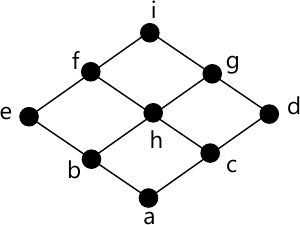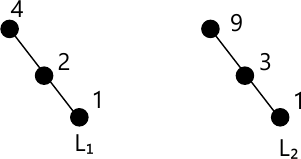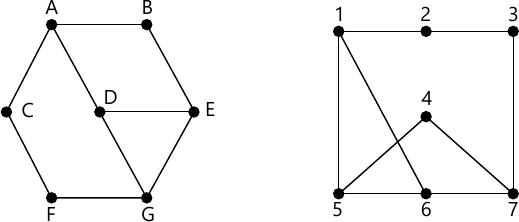MORE IN Discrete Structures
MU Computer Engineering (Semester 3)
Discrete Structures
December 2015
Total marks: --
Total time: --
INSTRUCTIONS
(1) Assume appropriate data and state your reasons
(2) Marks are given to the right of every question
(3) Draw neat diagrams wherever necessary

1 (a) Show that if any seven points are chosen in a regular hexagon whose sides are of 1 unit, then two of them must be no further apart than 1 unit.
5 M
1 (b) Determine the number of edges in a graph with 6 nodes, 2 nodes of degree 4 and 4 nodes of degree 2. Draw two such graphs.
5 M
1 (c) 6n+2+72n+1 is divisible by 43.
5 M
1 (d) Draw the Hasse diagram of D60. Also find whether it is a lattice.
5 M

2 (a) Define injective, surjective and bijective functions. if f: R→R and g: R→R defined by f(x)=x+2 and g(x)=x2. Find i) f⚬g⚬f ii) g⚬f⚬g
6 M
2 (b) It was found that in a class, 80 students are passed in English, 60 in Science and 50 in Mathematics. It was also found that 30 students passed in both English and Science, 15 students passed in both English and Mathematics and 20 students passed in both Mathematics and Science, 10 Students passed in all three subjects. If there are 150 students in the class, find
i) How many students passed in at least one subject?
ii) How many students passed in English only?
How many students failed in all three subjects?
8 M
2 (c) Let S={1, 2, 3, 4, 5} and A=S×S. Define the following relation R on A: (a,b) R(c,d) if and only if ad=bc show that R is an equivalence relation and compute A/R.
6 M

3 (a) If 11 people are chosen from a set of A={1, 2, 3, ....., 20}, then one of them is multiple of other.
4 M
3 (b) If f:A→B and if g: B→C are both one-one and onto. then (g⚬f)-1 = f-1⚬g-1.
4 M
3 (c) Determine whether the below Hasses diagram represents a lattice.6 M
3 (d) If (G, *) is an Abelian group, then for all a, b ∈ G show that (a*b)n = an+bn. (Use mathematical induction).
6 M

4 (a) Let G={1,2,3,4,5,6}. Prove that (G, X7) is a finite Abelian group with respect to multiplication modulo 7.
6 M
4 (b) Let H$$=\begin{bmatrix} 1 &0 &0 \\1 &1 &0 \\0 &1 &1 \\1 &0 &0 \\0 &1 &0 \\0 &0 &1 \end{bmatrix}$$ be parity check matrix.
Determine the group code eH: B2→B5.
7 M
4 (c) Find the generation functions for the following sequence
i) 0, 0, 0, 1, 2, 3, 4, 5, 7, ..........
ii) 6, -6, 6, -6, 6, -6, 6, -...........
7 M

5 (a) If function f is an isomorphism from semigroup (S, *) to (T, *'), then prove that f-1 is an isomorphism from (T, *') to (S, *).
5 M
5 (b) Consider the chain of divisors of 4 and 9, i.e. L1={1, 2, 4} and L2={1, 3, 9}
Find the Hasse diagram of L1×L2.7 M
5 (c) Show that the set G={f1, f2, f3, f4, f5, f6} where the function are defined by $f_1(x)=x, \ \ f_2(x)= 1-x, \ \ f_3(x) = \dfrac {x}{x-1} \\ f_4(x)= \dfrac {1}{x} \ \ f_5 = \dfrac {1}{1-x} \ \ f_6(x) = 1 - \dfrac {1}{x}$ is a group under composition of functions. Frame the composite table.
8 M

6 (a) Determine whether following graphs are isomorphic8 M
6 (b) Solve the following recurrence relation: an-5an-1+6an-2=2n with initial conditions a0=-1 and a1=1.
7 M
6 (c) Show that $$\big (\neg \text q \wedge(\text p \Rightarrow \text q)\big ) \Rightarrow \neg \text p$$ is a tautology.
5 M

More question papers from Discrete Structures